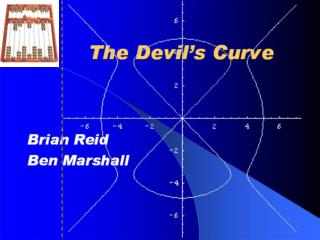DownloadDownload PresentationThe Devil’s Curve

# The Devil’s Curve

Télécharger la présentation## The Devil’s Curve

- - - - - - - - - - - - - - - - - - - - - - - - - - - E N D - - - - - - - - - - - - - - - - - - - - - - - - - - -
##### Presentation Transcript

1. The Devil’s Curve Brian Reid Ben Marshall

2. History of the Devil’s Curve • 1750, First investigated by Gabriel Cramer (1704-1752) • Cramer’s Rule • 1810, studied by Lacroix • First appeared in Nouvelles Annales 1858 • “Devil” name comes from diabolo game (Italy)

3. Equations • Cartesian

4. Constant “a”  linear distortion • B=25/24 “electric motor curve” • Center of curve shows coil of a wire , which rotates by forces exerted by surrounding magnets • Crunode at the origin • When a/b>1  vertical • a/b<1  horizontal • a=b  circle

5. t=linspace(0,pi,60);a=1;b=sqrt(2);x=cos(t).*sqrt((a^2.*sin(t).^2-b^2.*cos(t).^2)./(sin(t).^2-cos(t).^2));y=sin(t).*sqrt((a^2.*sin(t).^2-b^2.*cos(t).^2)./(sin(t).^2-cos(t).^2));plot(real(x),real(y))hold ont=linspace(0,-pi,60);a=1;b=sqrt(2);x=cos(t).*sqrt((a^2.*sin(t).^2-b^2.*cos(t).^2)./(sin(t).^2-cos(t).^2));y=sin(t).*sqrt((a^2.*sin(t).^2-b^2.*cos(t).^2)./(sin(t).^2-cos(t).^2));plot(real(x),real(y))grid onaxis equalxlabel('x axis'),ylabel('y axis')

6. FIN.